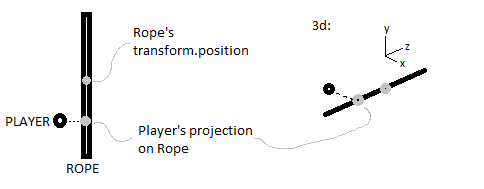# Vector3.Project, how to?

Hello, I’m trying to make my character to be placed on a rope when coming near to it.
I’ve done everything now, the only problem, is placing the character on the exact X and Z location ( the Y position is the same ).
The solution seems to be using a projection of my character on the rope, and then place the character on this projection, but I don’t know exactly how to do this.

Here’s the exemple:the rope’s transform.position is in the center of the rope, And its transform.forward points where the rope mesh points. What I want is to get an axis from the rope, and then get the projection of my player on it.
Can someone help me with this?

It’s pretty easy. You create a vector(V1) from your rope transform (blue dot) to your player (red dot). As normal vector you use the rope-transform-forward vector (i guess your line is along the forward vector). Since .forward is already normalized you don’t have to normalize it.

then just insert V1 and as normal .forward into Vector3.Project and you get the vector(V2) from the ropeT (blue) to your desired point P (yellow).

Finally you just have to add the rope transform position and the projected vector V2 together and you get the world position of the point P.An example:

``````//C#
public Transform rope;
public Transform player;

Vector3 ProjectPointOnRope(Vector3 aPoint)
{
Vector3 V1 = aPoint - rope.position;
Vector3 V2 = Vector3.Project(V1, rope.forward);
return rope.position + V2;
}

void Test()
{
Vector3 projectedPlayer = ProjectPointOnRope(player.position);
// ...

}
``````

In one line it would be:

``````// C# and UnityScript:
var projectedPlayer = rope.position + Vector3.Project(player.position - rope.position, rope.forward);
``````

edit

The above method could be generalized as extension method for the Ray struct:

``````public static Vector3 Project(this Ray aRay, Vector3 aPoint)
{
Vector3 V1 = aPoint - aRay.origin
Vector3 V2 = Vector3.Project(V1, aRay.direction);
return aRay.origin + V2;
}
``````

This would allow you to project any point onto the given ray:

``````var ray = new Ray(rope.position, rope.forward);
var projectedPlayer = ray.Project(player.position);
``````

You can get the position on the rope by intersecting the line that is the rope with a plane facing up at the correct ‘y’ height. Take a look at the Wiki Math3D functions:

http://wiki.unity3d.com/index.php/3d_Math_functions

In particular look at LinePlaneIntersection(). The plane normal with be Vector3.up, the plane point with be Vector3(0,y,0), the line point will be rope.position, and the line vector will be rope.up.

Note this will only work if you are guaranteed that rope exist at the specified ‘y’ height. If there is any chance that the player will be below or above the rope, then you can use Unity’s mathematical Plane class and raycast from each end of the rope to generate the position and figure out if the rope exists at that specific height. Or you can just check the ‘y’ height of each end of the rope after doing the line/plane intersection calculation.

There are computationally more efficient ways to do this calculation, but unless you are going to be doing it many times a frame, probably not worth the effort to code them.